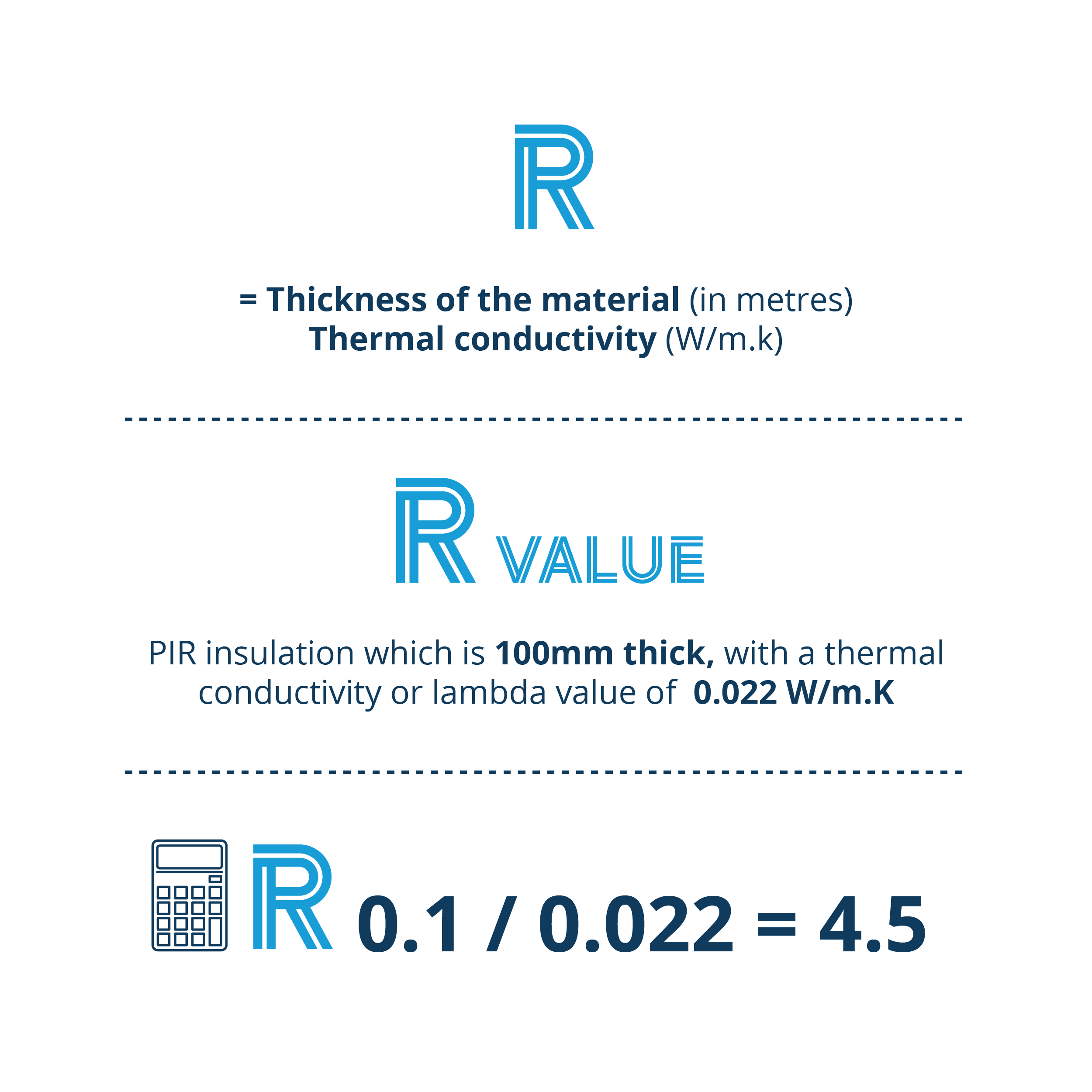# R-Values

In the UK construction industry, an “R-value” is often quoted.  This is a measure of thermal resistance. The R-value takes both the lambda value of a material and the actual thickness into account. Meeting very high R-values at minimal thicknesses is a crucial factor in reducing the amount of materials used in energy efficient buildings.

As an example, if you take a PIR insulation product which is 100mm thick, with a thermal conductivity or lambda value of  0.022 W/m.K, you would divide the thickness by the thermal conductivity by giving an R-value of 4.5.

An R-value shows how good a material is at resisting the flow of heat. If it resists the flow of heat well (has a high R-value) then it will be a better performing insulating material.  The higher the R-value of an insulation product, the better.

The R-value of the individual components of a building element such as a wall becomes important when choosing which insulation to use and in making a U-value calculation.